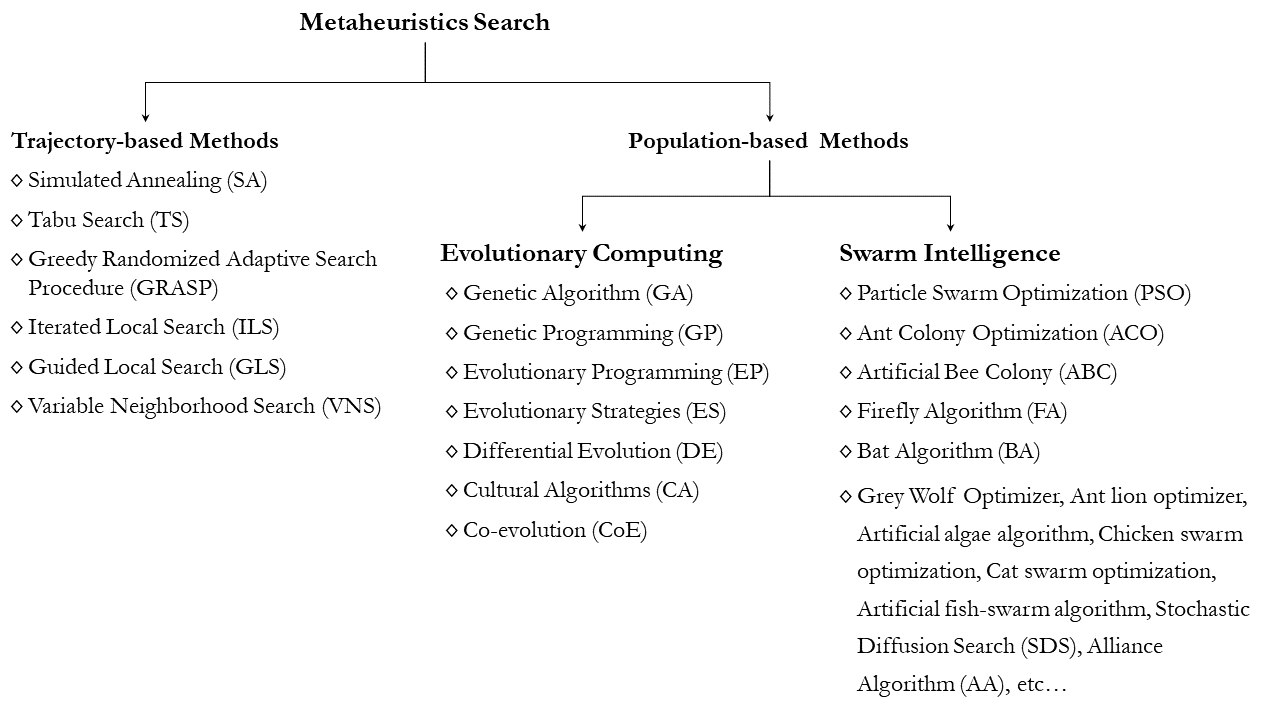# Trajectory-based Algorithms#

Metaheuristic algorithms can be classified into trajectory-based and population-based algorithms. A trajectory-based metaheuristic algorithm such as simulated annealing use a single agent or solution which moves through the design space or search space in a piecewise style. A better move or solution is always accepted, while a not-so-good move can be accepted with certain probability. The steps or moves trace a trajectory in the search space, with a non-zero probability that this trajectory can reach the global optimum. On the other hand, population-based algorithms such as genetic algorithms, ant colony optimization and particle swarm optimization use multiple agents to search for an optimal or near-optimal solution.A trajectory-based metaheuristic algorithm such as simulated annealing use a single agent or solution which moves through the design space or search space in a piecewise style. A better move or solution is always accepted, while a not-so-good move can be accepted with certain probability. The steps or moves trace a trajectory in the search space, with a non-zero probability that this trajectory can reach the global optimum.

Two algorithms are covered in this section: